# Sources and descriptions of datasets

This R notebook explores the Ontario Works (social assistance for people without income) case numbers in the dataset Head of Household from Toronto Employment and Social Services. We compute mean monthly rates for a given year (2013, the latest year with data for all months), and plot this data as maps of Toronto divided into wards (44 wards) and neighbourhoods (140 neighbourhoods).

https://www.toronto.ca/city-government/data-research-maps/open-data/open-data-catalogue/community-services/#15580d71-31ca-010e-3895-6b77b49d966f

This dataset contains the Head of Household trending data from 2004 to present. It provides information on households by neighbourhood with reference to the Ontario Works social assistance program. The information in this dataset will inform the delivery of services by providing trending information on social assistance.

Currency: March 2015

Children, Community and Social Services, Ontario, Social Assistance Caseloads

Total numbers of social assistance recipients and beneficiaries each month since January 1969.

https://files.ontario.ca/opendata/historical_sa_recipients_dataset_q2_2018_19.xlsx

Maytree, Social Assistance Summaries:

The Social Assistance Summaries series tracks the number of recipients of social assistance (welfare payments) in each province and territory.

Income of individuals by age group, sex and income source, Canada, provinces and selected census metropolitan areas

Table: 11-10-0239-01 (formerly CANSIM 206-0052)

“1110023901-noSymbol.csv”

“Number of persons” = number of persons whose age is \(\geq 16\).

“Number with income” = number of persons with income from social assistance

# Reading datasets and grouping observations

``````library(data.table)
library(plyr) #join
library(ggplot2) #fortify, ggplot
library(scales) #scale_fill_distiller
library(sp) #used by rgdal
library(ggmap) #theme_nothing
library(rgeos) #gCentroids
library(forecast) #auto.arima, forecast``````

Read .csv containing monthly TESS Ontario works cases for January 2004 to November 2014. The size of the csv file is 2.68 GB.

``````CSVFILE <- "opendata_tess_ow.csv"
``````|--------------------------------------------------|
|==================================================|``````

Structure of datatable:

``str(tess_dt)``
``````Classes ‘data.table’ and 'data.frame':  9536341 obs. of  21 variables:
\$ YEAR_NUM              : int  2004 2004 2004 2004 2004 2004 2004 2004 2004 2004 ...
\$ MNTH                  : int  20040101 20040101 20040101 20040101 20040101 20040101 20040101 20040101 20040101 20040101 ...
\$ PROGRAM_NM            : chr  "Ontario Works" "Ontario Works" "Ontario Works" "Ontario Works" ...
\$ OFFICE                : chr  "Application Centre" "Application Centre" "Application Centre" "Application Centre" ...
\$ FAMILY_TYP_NM         : chr  "Families" "Families" "Families" "Families" ...
\$ FAMILY_SIZE           : chr  "2" "2" "2" "2" ...
\$ AGE                   : chr  "18 to 29 yrs old" "18 to 29 yrs old" "18 to 29 yrs old" "30 to 39 yrs old" ...
\$ EDUCATION_LEVEL       : chr  "High School Incomplete" "High School Incomplete" "Post Secondary" "High School Complete" ...
\$ EARNINGS              : chr  "" "" "" "Earnings from employment" ...
\$ IMMIGRATION_STATUS    : chr  "Permanent Resident" "Permanent Resident" "Canadian Citizen" "Canadian Citizen" ...
\$ TIMES_ON_ASSISTANCE   : chr  "1" "4+" "3" "1" ...
\$ MONTHS_ON_ASSISTANCE  : chr  "1 to 6 months" "1 to 6 months" "1 to 6 months" "1 to 6 months" ...
\$ MONTHS_OFF_ASSISTANCE : chr  "1 to 6 months" "1 to 6 months" "1 to 6 months" "7 to 24 months" ...
\$ GENDER                : chr  "F" "F" "F" "F" ...
\$ SHELTER_COSTS         : chr  "\$600 to \$999" "\$400 to \$599" "\$200 to \$399" "\$400 to \$599" ...
\$ YOUNGEST_DEP_AGE_RANGE: chr  "less than 5 yrs old" "less than 5 yrs old" "less than 5 yrs old" "5 to 10 yrs old" ...
\$ WARD_SCODE            : int  2 2 8 8 6 2 1 2 8 6 ...
\$ CENSUS_NEIGH_SCODE    : int  4 4 24 27 19 4 2 4 27 19 ...
\$ NEW_CASES             : int  0 0 0 0 0 0 0 0 0 0 ...
\$ EXITS                 : int  1 0 0 0 1 0 0 0 0 0 ...
\$ CASES                 : int  1 1 1 1 1 1 1 1 1 1 ...
- attr(*, ".internal.selfref")=<externalptr> ``````

Select columns from tess_dt

``ow_cases <- tess_dt[,.(YEAR_NUM, MNTH, WARD_SCODE, CENSUS_NEIGH_SCODE, CASES)]``

Drop rows where at least one of CENSUS_NEIGH_SCODE and WARD_SCODE is missing

``ow_cases_dropna <- na.omit(ow_cases, cols=c("CENSUS_NEIGH_SCODE", "WARD_SCODE"))``

Calculate mean number of cases in 2010 by month and ward

``````ow_wards_2010 <- ow_cases_dropna[YEAR_NUM==2010,.(TOTAL_CASES=sum(CASES)),by=.(MNTH, WARD_SCODE)]

ow_wards_2010_months <- ow_wards_2010[,.(MONTHLY_CASES=sum(TOTAL_CASES)),by=.(MNTH, WARD_SCODE)]

ow_wards_2010_monthly <- ow_wards_2010_months[,.(MEAN_CASES_PER_MONTH=mean(MONTHLY_CASES)),by=.(WARD_SCODE)]``````

``ow_wards_2010_monthly\$id <- ow_wards_2010_monthly\$WARD_SCODE``

Calculate mean number of cases in 2013 by month and ward

``````ow_wards_2013 <- ow_cases_dropna[YEAR_NUM==2013,.(TOTAL_CASES=sum(CASES)),by=.(MNTH, WARD_SCODE)]

ow_wards_2013_months <- ow_wards_2013[,.(MONTHLY_CASES=sum(TOTAL_CASES)),by=.(MNTH, WARD_SCODE)]

ow_wards_2013_monthly <- ow_wards_2013_months[,.(MEAN_CASES_PER_MONTH=mean(MONTHLY_CASES)),by=.(WARD_SCODE)]``````

``ow_wards_2013_monthly\$id <- ow_wards_2013_monthly\$WARD_SCODE``

# Plotting data by wards

City Wards

Owner: City Clerk’s Office

Currency: August 2018

44 Ward Model - May 2010 (WGS84 - Latitude / Longitude)

http://opendata.toronto.ca/gcc/wards_may2010_wgs84.zip

Shapefile: icitw_wgs84

Wards: There are a total of 44 electoral wards in the City of Toronto
* GEO_ID = unique geographic identifier
* NAME = Name of the Ward with corresponding ward number
* SCODE_NAME = Ward Number
* LCODE_NAME = Ward Number and the community council area it is in (N,S, E or W)
* TYPE_DESC = Ward
* TYPE_CODE = City Ward

We shall input wards shapefile, create centroids of wards, and process shapefile as dataframe. First we input the shapefile

``wards.sh <- readOGR("C:/Users/14165/Desktop/ArcGIS/SHAPEFILES/May2010_WGS84", "icitw_wgs84")``
``````OGR data source with driver: ESRI Shapefile
Source: "C:\Users\14165\Desktop\ArcGIS\SHAPEFILES\May2010_WGS84", layer: "icitw_wgs84"
with 44 features
It has 10 fields``````

``wards.sh@data\$id <- as.integer(wards.sh@data\$SCODE_NAME)``

Make centroids of each ward, for placing labels when plotting

``wards.sh.centroids  <- as.data.frame(gCentroid(wards.sh, byid = TRUE))``

``wards.sh.centroids\$id <- wards.sh@data\$id``

Shapefile processing

``````wards.sh.points = fortify(wards.sh, region="id")
wards.sh.df = join(wards.sh.points, wards.sh@data, by="id")``````

Merge ward shapefile and ow_wards_2010_monthly dataframe

``wards.sh.ow_2010 <- merge(wards.sh.df, ow_wards_2010_monthly, by = "id")``

Make graphics object for wards.sh.ow_2010

``````p.wards_2010 <- ggplot() +
geom_polygon(data = wards.sh.ow_2010,
aes(x = long, y = lat, group = group, fill = MEAN_CASES_PER_MONTH),
color = "black", size = 0.25) +
coord_map() +
scale_fill_distiller(name="Cases", palette = "YlOrBr", trans = "reverse", breaks = pretty_breaks(n = 8)) +
theme_nothing(legend = TRUE) +
labs(title="Mean monthly number of Ontario Works cases by ward in Toronto in 2010") +
geom_text(aes(x=x, y=y, group=NULL, label=id), data = wards.sh.centroids, size = 2)``````

Plot graphics object

``p.wards_2010 + guides(fill = guide_legend(reverse = TRUE)) #ggplot2``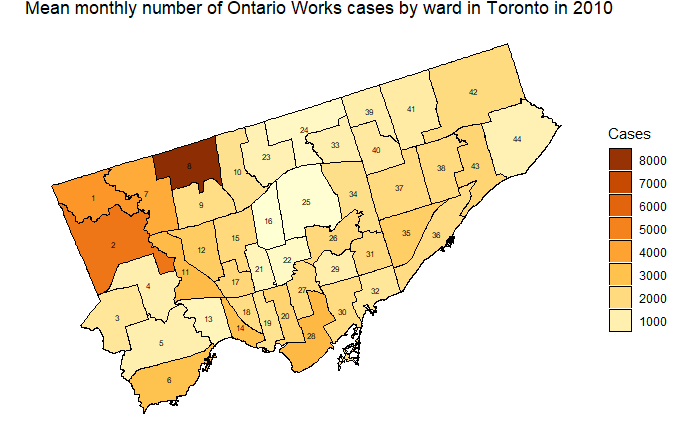Calculate mean number of cases in 2013 by month and ward

``````ow_wards_2013 <- ow_cases_dropna[YEAR_NUM==2013,.(TOTAL_CASES=sum(CASES)),by=.(MNTH, WARD_SCODE)]

ow_wards_2013_months <- ow_wards_2013[,.(MONTHLY_CASES=sum(TOTAL_CASES)),by=.(MNTH, WARD_SCODE)]

ow_wards_2013_monthly <- ow_wards_2013_months[,.(MEAN_CASES_PER_MONTH=mean(MONTHLY_CASES)),by=.(WARD_SCODE)] ``````

``ow_wards_2013_monthly\$id <- ow_wards_2013_monthly\$WARD_SCODE``

Merge ward shapefile and ow_wards_2013_monthly dataframe

``wards.sh.ow_2013 <- merge(wards.sh.df, ow_wards_2013_monthly, by = "id")``

Make graphics object

``````p.wards_2013 <- ggplot() +
geom_polygon(data = wards.sh.ow_2013,
aes(x = long, y = lat, group = group, fill = MEAN_CASES_PER_MONTH),
color = "black", size = 0.25) +
coord_map() +
scale_fill_distiller(name="Cases", palette = "YlOrBr", trans = "reverse", breaks = pretty_breaks(n = 8)) +
theme_nothing(legend = TRUE) +
labs(title="Mean monthly number of Ontario Works cases by ward in Toronto in 2013") +
geom_text(aes(x=x,y=y, group=NULL, label=id), data = wards.sh.centroids, size=2)``````

Plot graphics object

``p.wards_2013 + guides(fill = guide_legend(reverse = TRUE)) #ggplot2``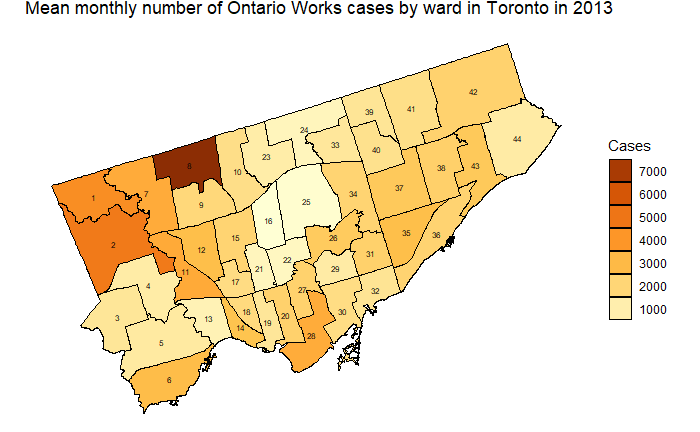Calculate mean number of cases in 2013 by month and neighbourhood

``````ow_nbds_2013 <- ow_cases_dropna[YEAR_NUM==2013,.(TOTAL_CASES=sum(CASES)),by=.(MNTH, CENSUS_NEIGH_SCODE)]

ow_nbds_2013_months <- ow_nbds_2013[,.(MONTHLY_CASES=sum(TOTAL_CASES)),by=.(MNTH, CENSUS_NEIGH_SCODE)]

ow_nbds_2013_monthly <- ow_nbds_2013_months[,.(MEAN_CASES_PER_MONTH=mean(MONTHLY_CASES)),by=.(CENSUS_NEIGH_SCODE)]``````

``ow_nbds_2013_monthly\$id <- ow_nbds_2013_monthly\$CENSUS_NEIGH_SCODE``

Doing finer aggregation: mean monthly cases who are single (without dependents)

``````ow_singles <- tess_dt[FAMILY_TYP_NM=="Singles",.(YEAR_NUM, MNTH, WARD_SCODE, CENSUS_NEIGH_SCODE, CASES)]

ow_singles_dropna <- na.omit(ow_singles, cols=c("CENSUS_NEIGH_SCODE", "WARD_SCODE"))

ow_singles_wards_2013 <- ow_singles_dropna[YEAR_NUM==2013,.(TOTAL_CASES=sum(CASES)),by=.(MNTH, WARD_SCODE)]

ow_singles_wards_2013_months <- ow_singles_wards_2013[,.(MONTHLY_CASES=sum(TOTAL_CASES)),by=.(MNTH, WARD_SCODE)]

ow_singles_wards_2013_monthly <- ow_singles_wards_2013_months[,.(MEAN_CASES_PER_MONTH=mean(MONTHLY_CASES)),by=.(WARD_SCODE)]``````

``ow_singles_wards_2013_monthly\$id <- ow_singles_wards_2013_monthly\$WARD_SCODE``

Merge ward shapefile and Ontario Works dataframe

``wards.sh.ow_singles_2013 <- merge(wards.sh.df, ow_singles_wards_2013_monthly, by = "id")``

We shall plot wards.sh.ow_singles_2013. Make graphics object

``````p.wards_singles_2013 <- ggplot() +
geom_polygon(data = wards.sh.ow_singles_2013,
aes(x = long, y = lat, group = group, fill = MEAN_CASES_PER_MONTH),
color = "black", size = 0.25) +
coord_map() +
scale_fill_distiller(name="Cases", palette = "PuRd", trans = "reverse", breaks = pretty_breaks(n = 8)) +
theme_nothing(legend = TRUE) +
labs(title="Mean monthly number of Ontario Works cases (singles) by ward in Toronto in 2013") +
geom_text(aes(x=x,y=y, group=NULL, label=id), data = wards.sh.centroids, size = 2)``````

Plot graphics object

``p.wards_singles_2013 + guides(fill = guide_legend(reverse = TRUE))``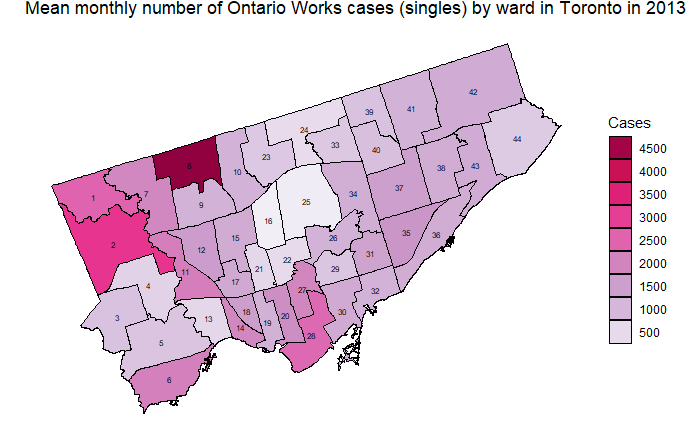# Plotting data by neighbourhoods

Neighbourhoods shapefile

https://www.toronto.ca/city-government/data-research-maps/open-data/open-data-catalogue/#a45bd45a-ede8-730e-1abc-93105b2c439f

http://opendata.toronto.ca/gcc/neighbourhoods_planning_areas_wgs84.zip

Owner: Social Development, Finance & Administration

Currency: June 2014

Neighbourhoods (WGS84)

Shapefile: NEIGHBORHOODS_WGS84

* Column name (Description)
* AREA_S_CD = AREA_SHORT_CODE
* AREA_NAME = AREA_NAME

Input shapefile

``nbds.sh <- readOGR("C:/Users/14165/Desktop/ArcGIS/SHAPEFILES/neighbourhoods_planning_areas_wgs84", "NEIGHBORHOODS_WGS84")``
``````OGR data source with driver: ESRI Shapefile
Source: "C:\Users\14165\Desktop\ArcGIS\SHAPEFILES\neighbourhoods_planning_areas_wgs84", layer: "NEIGHBORHOODS_WGS84"
with 140 features
It has 2 fields``````

``nbds.sh@data\$id <- as.integer(nbds.sh@data\$AREA_S_CD)``

Make centroids of each neighbourhood, for placing labels when plotting

``nbds.sh.centroids  <- as.data.frame(gCentroid(nbds.sh, byid = TRUE))``

``nbds.sh.centroids\$id <- nbds.sh@data\$id``

Shapefile processing

``````nbds.sh.points = fortify(nbds.sh, region = "id")
nbds.sh.df = join(nbds.sh.points, nbds.sh@data, by = "id")``````

Merge neighbourhood shapefile and Ontario Works dataframe

``nbds.sh.ow_2013 <- merge(nbds.sh.df, ow_nbds_2013_monthly, by = "id")``

Make graphics object

``````p.nbds_2013 <- ggplot() +
geom_polygon(data = nbds.sh.ow_2013,
aes(x = long, y = lat, group = group, fill = MEAN_CASES_PER_MONTH),
color = "black", size = 0.2) +
coord_map() +
scale_fill_distiller(name="Cases", palette = "YlOrBr", trans = "reverse", breaks = pretty_breaks(n = 8)) +
theme_nothing(legend = TRUE) +
labs(title="Mean monthly number of Ontario Works cases by neighbourhood in Toronto in 2013") +
geom_text(aes(x=x,y=y, group=NULL, label=id), data = nbds.sh.centroids, size = 2)``````

Plot graphics object

``p.nbds_2013+guides(fill = guide_legend(reverse = TRUE))``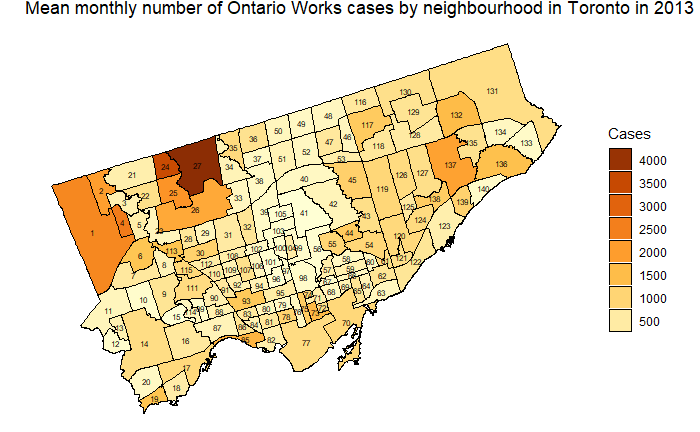# Time series

Avril Coghlan, Using R for Time Series Analysis

Vincent Zoonekynd, Time series

## TESS Ontario Works data manipulation

Group by month and create time series:

``````ow_cases_months <- tess_dt[,.(MNTH, CASES, NEW_CASES, EXITS)]

ow_cases_monthly <- ow_cases_months[,.(CASES=sum(CASES), NEW_CASES=sum(NEW_CASES), EXITS=sum(EXITS)),by=.(MNTH)]

ow_cases_monthly\$MNTH <- NULL

``````ow_ts <- ts(ow_cases_monthly, start = c(2004,1), frequency = 12)

``````         CASES NEW_CASES EXITS
Jan 2004 70169      3597  3788
Feb 2004 70327      3407  3977
Mar 2004 70929      5042  4430
Apr 2004 70040      5044  3917
May 2004 69999      4567  4146
Jun 2004 69976      4806  4318``````

## TESS Ontario Works plots

Plot time series

``````options(scipen=999) #do not use scientific notation

plot(ow_ts)``````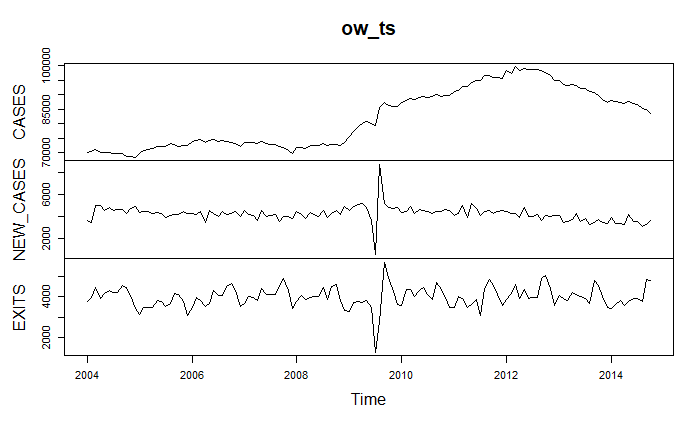Make time series of CASES

``````CASES <- ts(ow_cases_monthly\$CASES, start = c(2004,1), frequency = 12)

plot(CASES)``````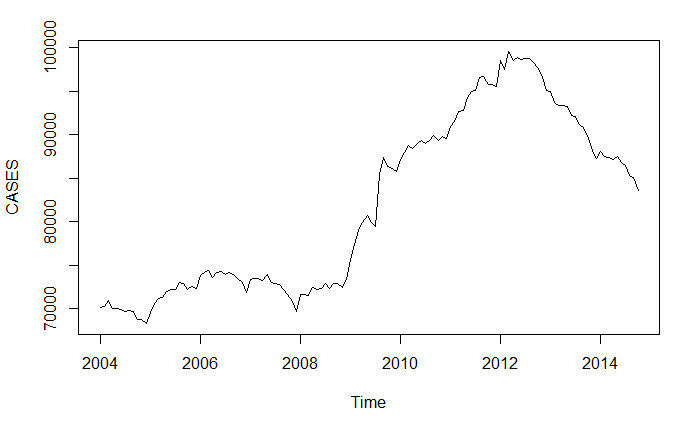Make time series of NEW_CASES

``````NEW_CASES <- ts(ow_cases_monthly\$NEW_CASES, start = c(2004,1), frequency = 12)

plot(NEW_CASES)``````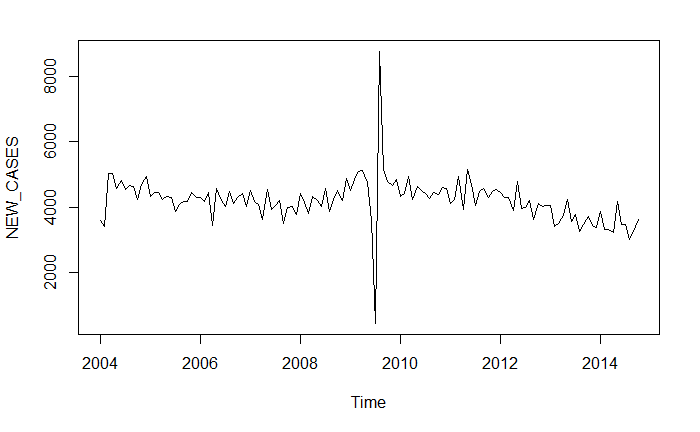## Decomposing time series into trend, seasonal, and remainder terms

Now we use stats::decompose and stats::stl to separate time series as a sum of trend, seasonal, and remainder terms.

``CASES_components <- decompose(CASES)``
``autoplot(CASES_components)``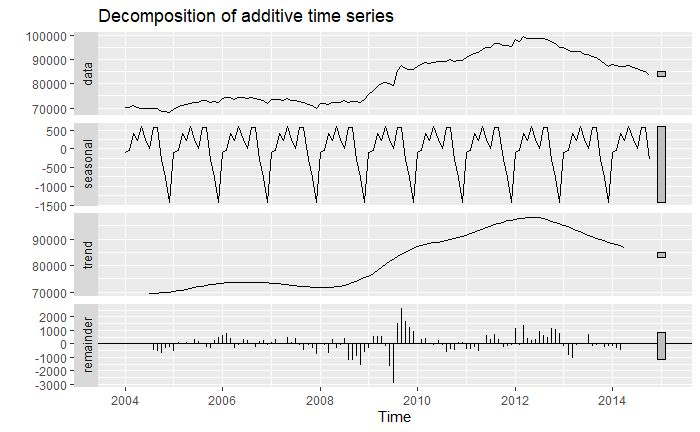``NEW_CASES_components <- decompose(NEW_CASES)``
``autoplot(NEW_CASES_components)``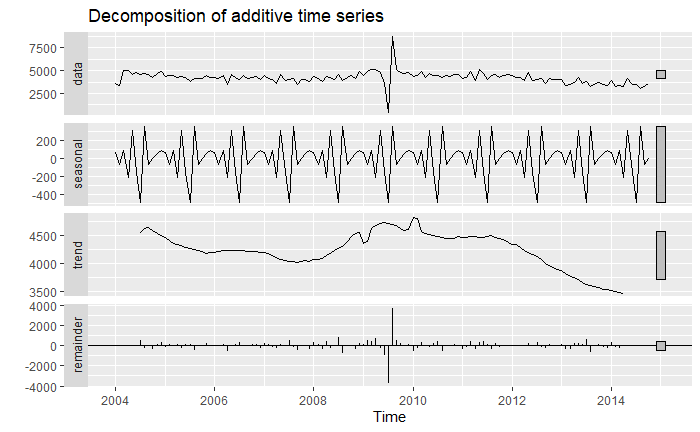``CASES.stl <- stl(CASES, s.window = "periodic")``
``autoplot(CASES.stl)``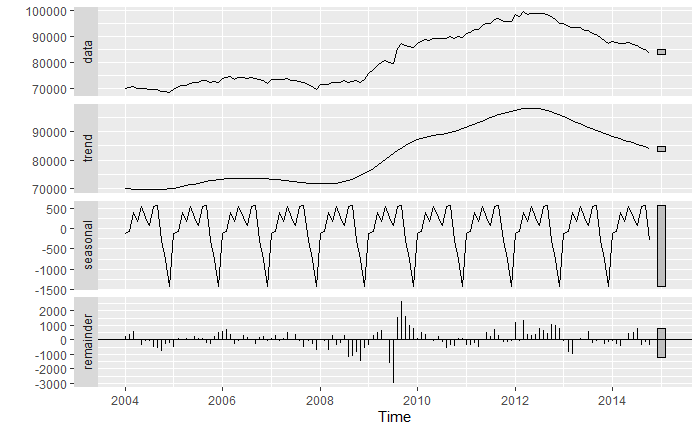``NEW_CASES.stl <- stl(NEW_CASES, s.window = "periodic")``
``autoplot(NEW_CASES.stl)``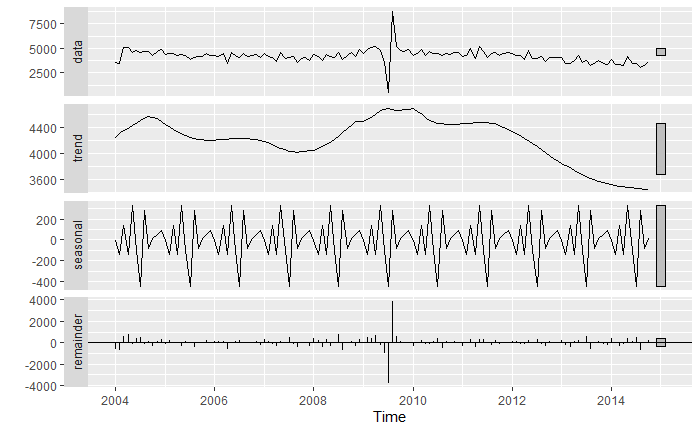## Holt-Winters exponential smoothing

``````CASES.HW <- HoltWinters(CASES)

CASES.HW``````
``````Holt-Winters exponential smoothing with trend and additive seasonal component.

Call:
HoltWinters(x = CASES)

Smoothing parameters:
alpha: 0.9535295
beta : 0.1484578
gamma: 1

Coefficients:
[,1]
a   84371.18862
b    -445.73297
s1  -1132.80119
s2  -1618.51347
s3   -369.32564
s4     -3.67365
s5    626.57812
s6    466.48438
s7    853.77241
s8    638.32040
s9    352.15093
s10   473.25854
s11   298.98513
s12  -793.18862``````
``plot(CASES.HW)``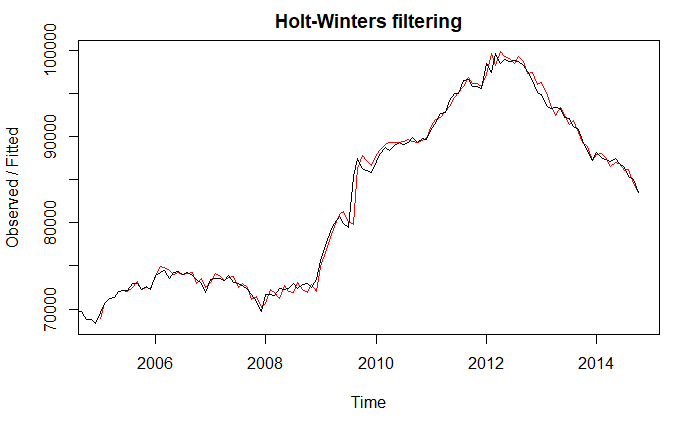``````CASES.HW.forecast <- forecast(CASES.HW, h = 24)

plot(CASES.HW.forecast)``````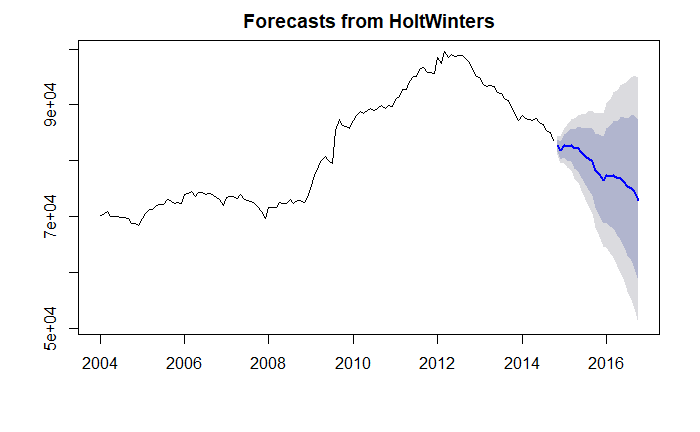## ARIMA

``CASES.arima <- auto.arima(CASES)``
``CASES.arima``
``````Series: CASES
ARIMA(0,2,1)(0,0,2)

Coefficients:
ma1    sma1    sma2
-0.8806  0.1925  0.2403
s.e.   0.0497  0.0852  0.0827

sigma^2 estimated as 825250:  log likelihood=-1053.56
AIC=2115.11   AICc=2115.44   BIC=2126.52``````
``````op <- par(mfrow=c(2,1), mar=c(2,4,1,2)+.1)
acf(CASES,  main="")
pacf(CASES, main="")``````
``par(op)``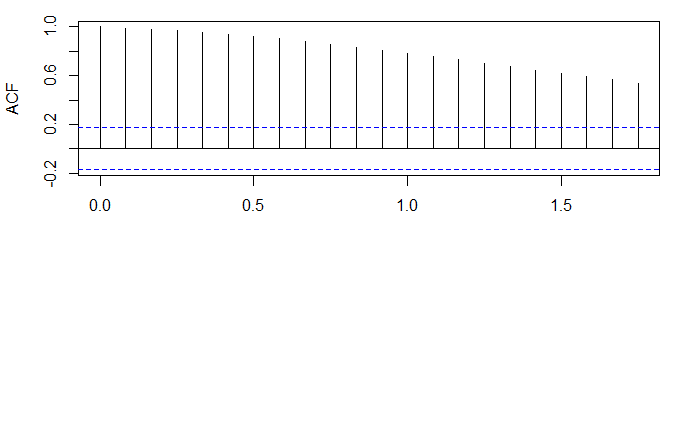``````CASES.forecast <- forecast(CASES, level = c(95), h = 22)

plot(CASES.forecast)``````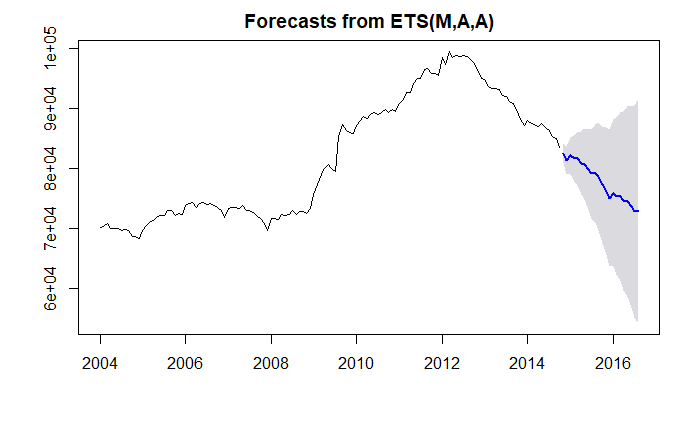``````CASES.arima.forecast <- forecast(CASES.arima, level = c(95), h = 22)

plot(CASES.arima.forecast)``````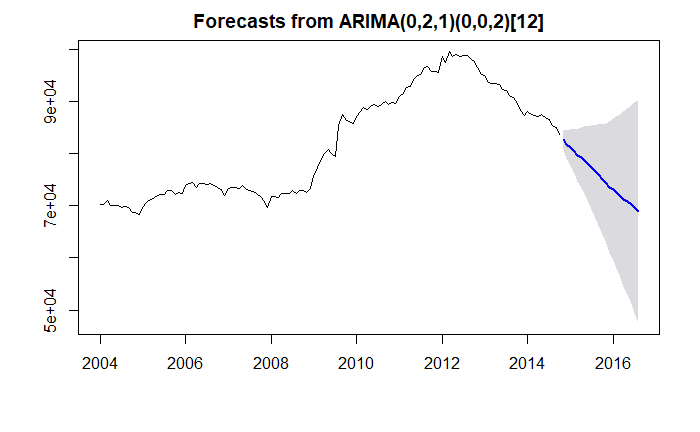## Ontario Monthly Social Assistance Caseloads 1969-2018

``````SA <- fread("historical_sa_recipients_dataset_q2_2018_19.csv")

SA\$Beneficiaries <- NULL``````

Make time series

``SA_ts <- ts(SA\$Cases, start = c(1969,1), frequency = 12)``
``plot(SA_ts)``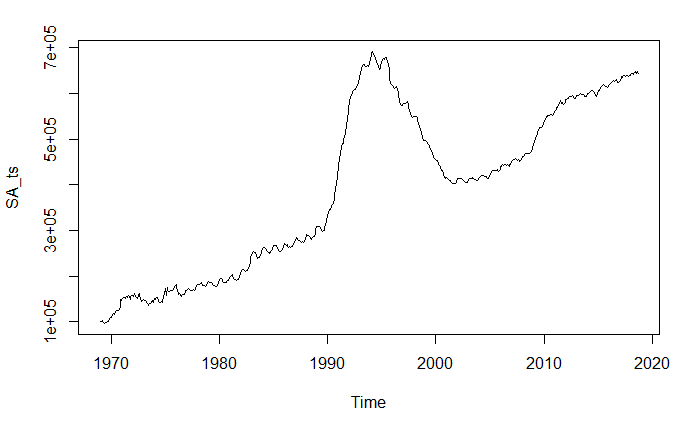``````SA_ts_1997 <- window(SA_ts, c(1997,1))

plot(SA_ts_1997)``````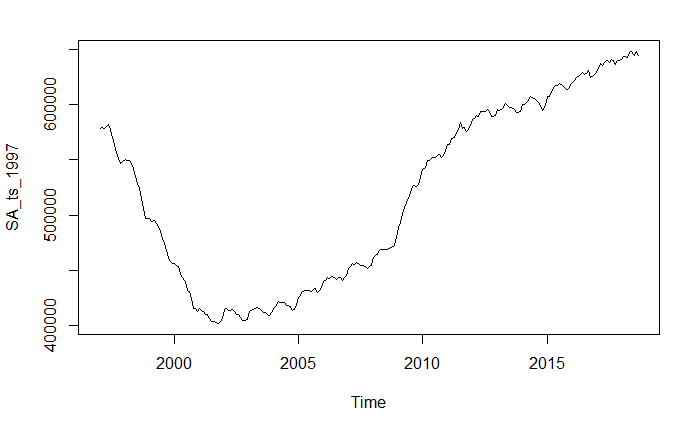``````SA_ts_1997.components <- decompose(SA_ts_1997)

autoplot(SA_ts_1997.components)``````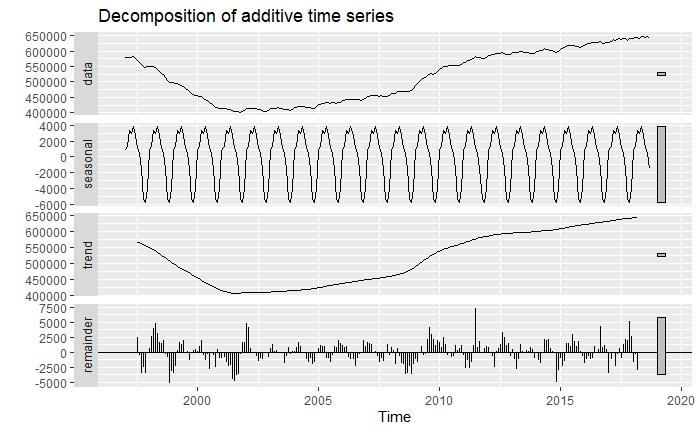``````SA_ts_1997.stl <- stl(SA_ts_1997, s.window = "periodic")

autoplot(SA_ts_1997.stl)``````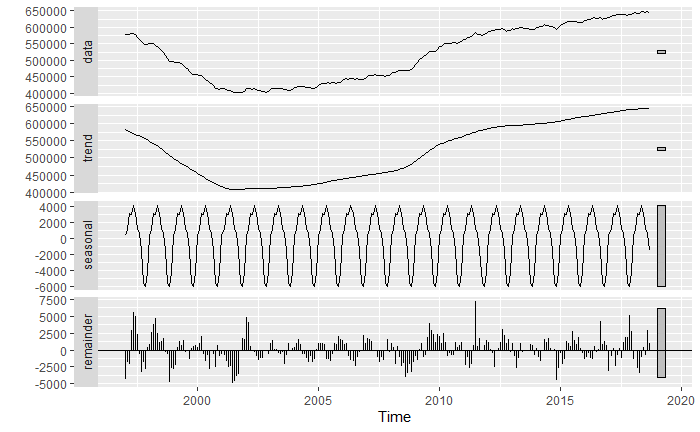## Maytree Social Assistance Summaries

``Maytree_ON <- fread("ON.csv")``
``str(Maytree_ON)``
``````Classes ‘data.table’ and 'data.frame':  25 obs. of  7 variables:
\$ Year                       : chr  "1997-98" "1998-99" "1999-00" "2000-01" ...
\$ Ontario Works Cases        : int  362334 310493 262439 215618 196596 195137 192096 191723 198377 199242 ...
\$ Ontario Works Beneficiaries: int  796109 690608 577620 469494 419493 404067 389754 380670 386801 383068 ...
\$ ODSP Cases                 : int  185479 189392 189536 191885 192048 194140 200087 205880 212058 221718 ...
\$ ODSP Beneficiaries         : int  261737 268159 268286 271144 270558 271740 278393 285231 292622 305202 ...
\$ Total Cases                : int  547813 499884 451975 407503 388644 389277 392183 397603 410435 420960 ...
\$ Total Beneficiaries        : int  1057846 958767 845907 740637 690051 675807 668148 665901 679423 688270 ...
- attr(*, ".internal.selfref")=<externalptr> ``````
``````Maytree_OW.ts <- ts(Maytree_ON\$`Ontario Works Cases`, start = 1997, frequency = 1)

Maytree_ODSP.ts <- ts(Maytree_ON\$`ODSP Cases`, start = 1997, frequency = 1)``````
``plot(Maytree_OW.ts)``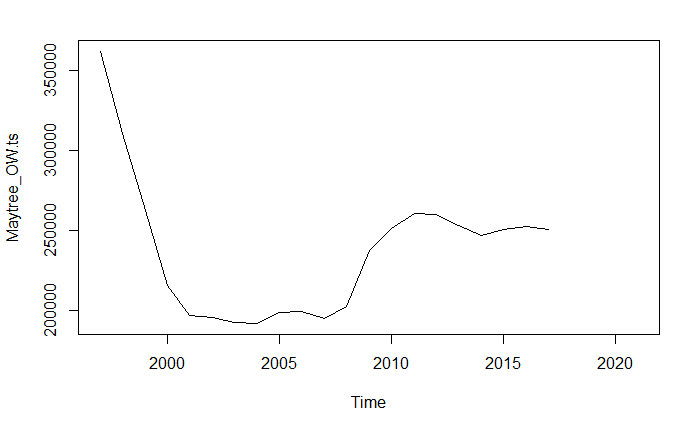``plot(Maytree_ODSP.ts)``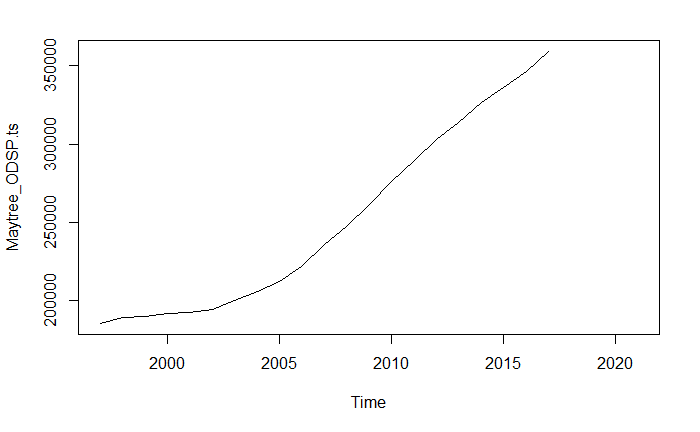``plot(Maytree_ODSP.ts+Maytree_OW.ts)``